Created October 22, 2017 © MIT

# Eye for visually impaired

Minimize the input of human assistance by introducing a system that can aid the visually impaired to perform everyday tasks.

IntermediateProtip20 hours139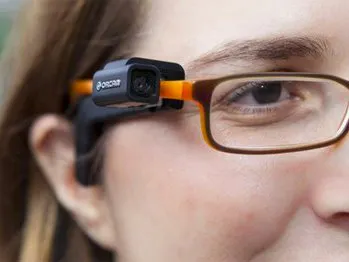## Things used in this project

### Hardware components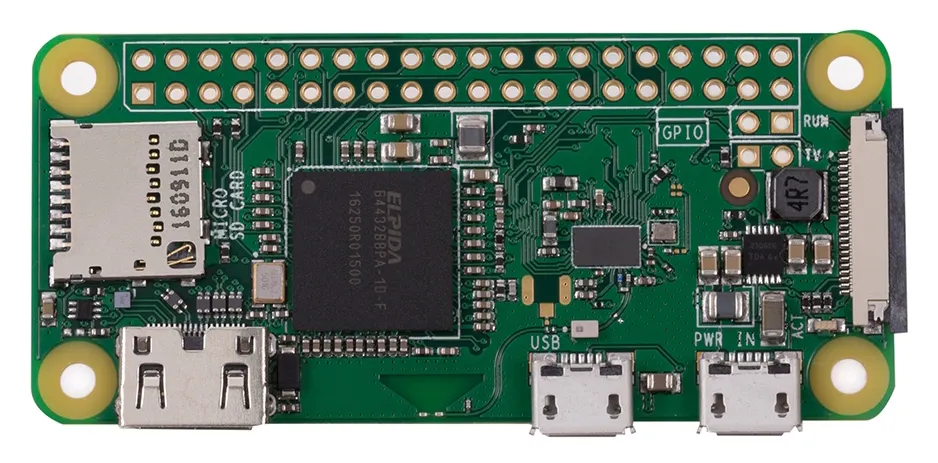Raspberry Pi Zero Wireless
×1Hologram Nova
×1Arduino Nano R3
×2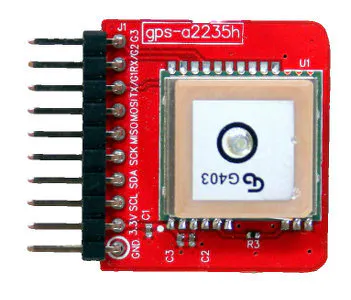Tessel GPS Module
×1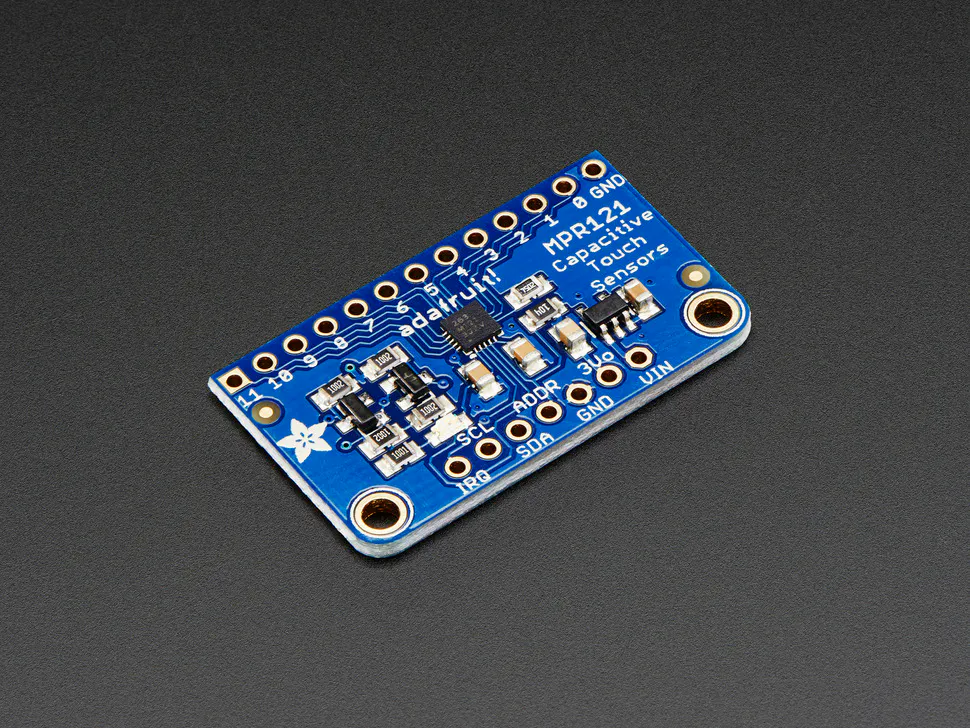Adafruit Capacitive Touch Sensor Breakout - MPR121
×1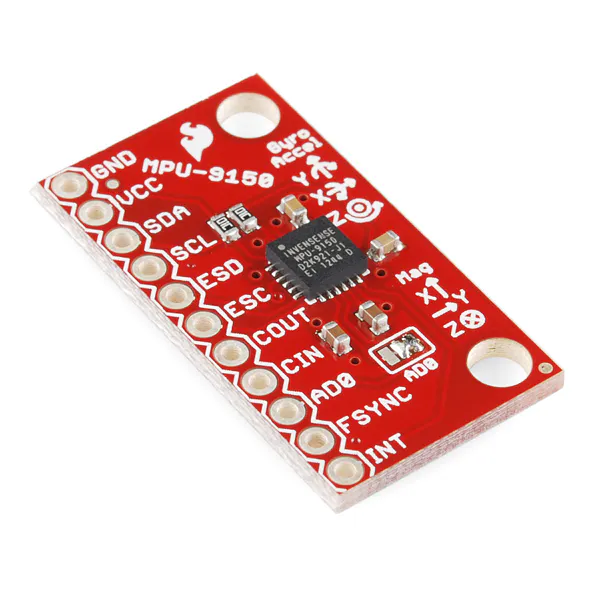SparkFun 9 Degrees of Freedom Breakout - MPU-9150
×2Raspberry Pi Camera Module
×1

### Software apps and online services### Hand tools and fabrication machinesSoldering iron (generic)

## Code

### Google maps API with instructions from TTS engine

Python
The code waits for user voice input for source place and the destination he/she would like to go. It then scraps the waypoints from source to destination and outputs a set of instructions for user to follow. This output is interpreted via TTS engine espeak.
```# Installed espeak TTS that is meant excuslively for python
# For installing : sudo apt-get install espeak python-espeak
# Needs only two line of code to make it work
# from espeak import espeak
# espeak.synth("String to be TTS converted")
# for changing property of voice refer to http://espeak.sourceforge.net/voices.html

import urllib
import urllib2
import xml.etree.ElementTree as ET
import math

import serial,time
ser=serial.Serial('/dev/ttyUSB0',baudrate=9600,timeout=None)

from espeak import espeak

api_key=""

user_set_origin=""
user_set_destination=""
mode=""             # This is user set mode
primary_data_dict={}
confirmed_data=""   # This data is user confirmed that can be used by other functions#

def stt_serial_input(str_output):
"A custom function for stt newline character #. Taken advantage of reading one byte"
print str_output
input_str=''
inchar=''

while inchar!='#':
input_str=input_str+inchar

input_str=input_str.replace('#','')
input_str=input_str.replace('*','')

print "Speech Recognised : ",input_str
return input_str

"function asks for user data and would return a list of it"
global user_set_origin
global user_set_destination
global mode

user_set_origin=""
user_set_destination=""
mode=""

user_defined_data_dict={}

while len(user_set_origin)<=1:
espeak.synth("Enter the origin, or beginning point. ")
user_set_origin=stt_serial_input("Enter the origin or beginning point:")
if len(user_set_origin)<=1:
print "This field cannot be left blank"
espeak.synth("This field cannot be left blank, ")
else:
user_defined_data_dict['USER_SET_ORIGIN']=user_set_origin
break

while len(user_set_destination)<=1:
espeak.synth("Enter the destination or the end point. ")
user_set_destination=stt_serial_input("Enter the destination or the end point:")
if len(user_set_destination)<=1:
print "This field cannot be left blank"
espeak.synth("This field cannot be left blank, ")
else:
user_defined_data_dict['USER_SET_DESTINATION']=user_set_destination
break

while 1:
espeak.synth("Enter the mode of transport, Available options are driving, bicycling, walking. ")
mode=stt_serial_input("Enter the mode of transport. Empty input defaults to driving. Available options driving, bicycling, walking:")
if len(mode)<=1:
mode="driving"
print "mode of transports defaults to driving"
espeak.synth("mode of transports defaults to driving. ")
user_defined_data_dict['USER_SET_MODE']=mode
break
elif mode=='driving' or mode=='bicycling' or mode=='walking':
print "Succesfully fetched mode:",mode
espeak.synth("Succesfully fetched mode, "+mode)
user_defined_data_dict['USER_SET_MODE']=mode
break
else:
print "Something went wrong. Its a typo or speech mistake. Enter data once again"
espeak.synth("Something went wrong. Its a typo or speech mistake. Enter data once again")
continue

return user_defined_data_dict

"This function would simply give raw_data as output that can be used by various function dependiing upon user input"
data=""
origin_api=user_dict['USER_SET_ORIGIN']
destination_api=user_dict['USER_SET_DESTINATION']
mode_api=user_dict['USER_SET_MODE']

url=website_comm+urllib.urlencode({'origin':origin_api,'destination':destination_api,'mode':mode_api,'region':'in','key':api_key})
handle=urllib2.urlopen(url)

for line in handle:
line=line.strip()
data=data+line

return data

def get_primary_data(raw_data):
"Function must give origin,destination and their co-ordinates as understood by api ( used for confirmation of data)"
"Also it would give total distance and time required to reach destination"
global confirmed_data
tree=ET.fromstring(raw_data)

primary_data_dict['start_gps_api_set']=(float(tree.find('.//leg/start_location/lat').text),float(tree.find('.//leg/start_location/lng').text))
primary_data_dict['end_gps_api_set']=(float(tree.find('.//leg/end_location/lat').text),float(tree.find('.//leg/end_location/lng').text))
primary_data_dict['total_distance']=long(tree.find('.//leg/distance/value').text)
primary_data_dict['total_duration']=tree.find('.//leg/duration/text').text

print "Please confirm the following details and proceed"
espeak.synth("Please confirm the following details and proceed")

time.sleep(2)

time.sleep(2)

print "Total time of travel is ",primary_data_dict['total_duration']

print "Total distance to destination :",primary_data_dict['total_distance']

time.sleep(1)
espeak.synth(" Confirm for the above printed data with Y (if yes) or N (if no).")

confirm_tag=stt_serial_input("Confirm for the above printed data with Y (if yes) or N (if no).")
if confirm_tag=='Y' or confirm_tag=='y' or confirm_tag=='' or confirm_tag=='yes':
confirmed_data=raw_data
return primary_data_dict

else:

def bearing_calculator(A,B): # A=(lat,lng) , B=(lat,lng) two orderes tuple
"gives bearing angle when looking from A towards B"

X=(math.cos(B))*(math.sin(B-A))
Y=(math.cos(A))*(math.sin(B))-(math.sin(A))*(math.cos(B))*(math.cos(B-A))

beta=(math.atan2(-X,Y)*180/math.pi)

if beta>=0:
return beta
else:
return beta+360

"This function would take in raw data and will give list of bearing angles that must be followed to reach target waypoint(steps)"
#lat_lng_lst=[]
bearing_data_lst=[]

for index,element in enumerate(gps_data_list):
if element==gps_data_list:
bearing_data_lst.append(bearing_calculator(primary_data_dict['start_gps_api_set'],element))
else:
bearing_data_lst.append(bearing_calculator(gps_data_list[index-1],element))

print bearing_data_lst
return bearing_data_lst

def get_steps_navigation(raw_data):     #must be given confirmed_data variable only
"This function would give steps and waythrough points and will output a list of lat & long : Exclusively for Anveshan project"
lat_lng_lst=[]                       #contains target waypoints. No start point
tree=ET.fromstring(raw_data)
#lat_long_tree_lst=tree.findall('.//step')

lat_lst=tree.findall('.//step/end_location/lat')
lng_lst=tree.findall('.//step/end_location/lng')

for x in range(0,len(lat_lst)):
lat_lng_lst.append((float(lat_lst[x].text),float(lng_lst[x].text)))

print lat_lng_lst